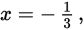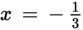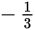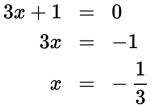# SAT Math Multiple Choice Question 298: Answer and Explanation

### Test Information

Question: 298

13. If p(x) is a polynomial function that has a simple zero at x = 4 and a double zero atwhich of the following could be the factored form of p(x)?

• A. p(x) = (x – 4)(x + 3)2
• B. p(x) = (x – 4)(3x + 1)2
• C. p(x) = 2(x – 4)(x + 3)
• D. p(x) = 2(x – 4)(3x + 1)

Explanation:

B

Difficulty: Medium

Category: Passport to Advanced Math / Exponents

Strategic Advice: A double zero occurs in a polynomial when a factor is repeated, or in other words, squared. For example, the factor (x - a) produces a simple zero at x = a, while (x - b)2 produces a double zero at x = b.

Getting to the Answer: The polynomial has a simple zero at x = 4, which corresponds to a factor of (x - 4), and all of the answers include this factor. The double zero atresults from a repeated (squared) factor, so you can eliminate C and D. To choose between A and (B), set each factor equal to 0 and then use inverse operations to solve for x (mentally if possible). The polynomial in A has zeroes at 4 and -3 (not), so you can eliminate A. Choice (B) is correct because it has a double zero when